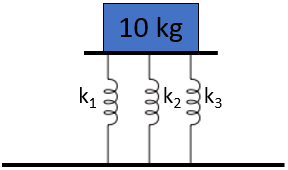## Parallel Springs

Consider three springs in parallel have the following spring constants (from left to right): 1 N/m, 2 N/m, & 3 N/m. If a 10 kg mass is placed on top, how many meters will the springs compress?Hint
The force in a spring is:
$$F_s=k\delta$$$where $$k$$ is the spring constant, and $$\delta$$ is the change in spring length from the un-deformed spring length. Hint 2 The spring constant for springs in parallel: $$k_{eq}=\sum_{i} k_i$$$
First, let’s determine the equivalent spring constant for several springs in parallel:
$$k_{eq}=\sum_{i} k_i$$$Since the problem statement has three springs in parallel: $$k_{eq}=k_1+k_2+k_3=1\frac{N}{m}+2\frac{N}{m}+3\frac{N}{m}=6\:N/m$$$
A spring’s deflection and force are related by:
$$F_s=k\delta$$$where $$F_s$$ is the forced applied to the spring, $$k$$ is the spring constant, and $$\delta$$ is the change in spring length from the un-deformed spring length. Before proceeding, we need to determine the spring force by multiplying the mass by gravity: $$F_s=mass\times acceleration=(10kg)(9.8m/s^2)=98\:N$$$
Now our units should cancel out when we solve for the spring’s compression distance:
$$\delta=\frac{F_s}{k_{eq}}=\frac{98N}{6N/m}=16.3\:m$$\$
16.3 m